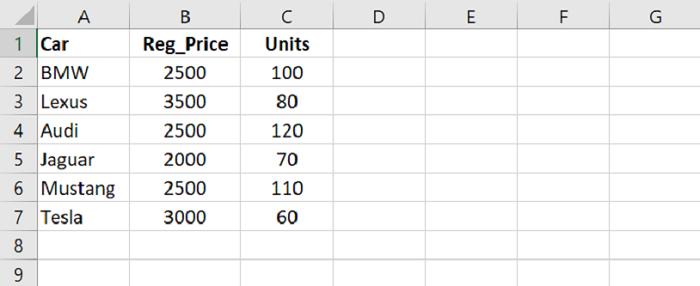# Rename column name with an index number of the CSV file in Pandas

Using columns.values(), we can easily rename column name with index number of a CSV file.

Let’s say the following are the contents of our CSV file opened in Microsoft Excel −We will rename the column names. At first, load data from a CSV file into a Pandas DataFrame −

dataFrame = pd.read_csv("C:\Users\amit_\Desktop\SalesData.csv")

Display all the column names from the CSV −

dataFrame.columns

Now, rename column names −

dataFrame.columns.values = "Car Names"
dataFrame.columns.values = "Registration Cost"
dataFrame.columns.values = "Units Sold"

## Example

Following is the code −

import pandas as pd

# Load data from a CSV file into a Pandas DataFrame:

# displaying column names
res = dataFrame.columns
# displaying another subset
print("\nDisplaying column names : \n",res)

# rename column names from the CSV file
dataFrame.columns.values = "Car Names"
dataFrame.columns.values = "Registration Cost"
dataFrame.columns.values = "Units Sold"

print("\nDisplaying updated column names : \n",res)

## Output

This will produce the following output −

Reading the CSV file...
Car   Reg_Price Units
0      BMW        2500 100
1    Lexus        3500 80
2     Audi        2500 120
3   Jaguar        2000 70
4  Mustang        2500 110

Displaying column names :
Index(['Car','Reg_Price','Units'], dtype='object')

Displaying updated column names :
Index(['Car Names','Registration Cost','Units Sold'], dtype='object')